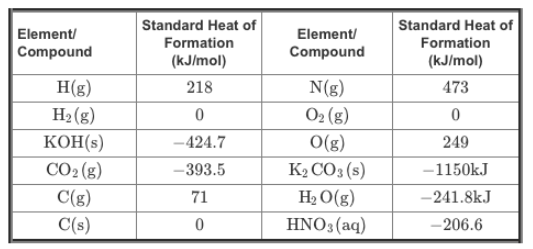# Problem: What is ΔH°rxn for the following chemical reaction? CO2(g) + 2 KOH (s) → H2O(g) + K2CO3(s) You can use the following table of standard heats of formation (ΔH°f) to calculate the enthalpy of the given reactionExpress the standard enthalpy of reaction to three significant figures and include the appropriate units

###### FREE Expert Solution

We can use the following equation to solve for ΔH˚rxn:

Note that we need to multiply each ΔH˚f by the stoichiometric coefficient since ΔH˚f is in kJ/mol.

Also, note that ΔH˚f for elements in their standard state is 0.

99% (122 ratings)###### Problem Details

What is ΔH°rxn for the following chemical reaction?

CO2(g) + 2 KOH (s) → H2O(g) + K2CO3(s)

You can use the following table of standard heats of formation (ΔH°f) to calculate the enthalpy of the given reactionExpress the standard enthalpy of reaction to three significant figures and include the appropriate units